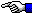# Four Fours:

## A Recreational Math Problem

This one is very simple: How many formulas can you devise, using only addition, subtraction, mulitplication and division, that are made up of only four digits of the number four that will produce as many numbers in the integer sequence 0, 1, 2, 3, 4, etc. as possible. (You can also use parentheses/brackets and let's also add SQUARE ROOTS too.) Here are some easy examples:

0 = (4-4)+(4-4)

and the easiest one for the integer 1 is:

`     441 = ----     44`

You must use a different formula for the integer 1 now. You should be able to find at least one formula for 1 through 10 before using a square root at all; so try that first.

Using square roots, you should be able to find formulas for every integer up through 20 (except for 19). For the integer 19, you are also allowed to use a decimal point in front of any of the four fours [.4] (that's 4/10 or 2/5, so something like: 4/.4 = 10 might give you an idea). An alternate method for arriving at a formula of four fours for 19 involves the symbol [!] which is interpreted as follows:

4! = 1 x 2 x 3 x 4 = 24.

The Starman.The Starman's Math Index